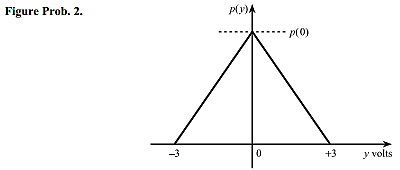A PCM transmitter sends out a 12-bit serial digital signal with 5 V corresponding to a 1 and 0 V corresponding to a 0. The signal passes over a transmission link, affected by random noise, to a PCM receiver consisting of a low-pass filter and a comparator. The comparator input signal is the PCM signal with noise superimposed on it; the probability density p(y) of this noise is the triangular function shown in Figure Prob. 2. The comparator decides that a 1 has been transmitted if the comparator input signal is greater than 2.5 V, and that a 0 has been transmitted if the comparator input signal is less than or equal to 2.5 V.

(a) Calculate p(0) such that p(y) is normalised.

(b) Find are probability that y is greater than +1.0 V.

(c) Find the probability that a 0 is received as a 1.

(d) Find the probability that a 1 is received as a 0.

(e) What is the probability that a single error occurs in a complete code word?